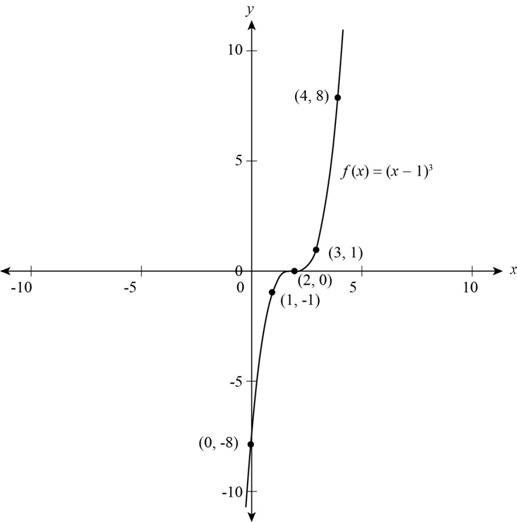# The formula that expresses f algebraically.### Precalculus: Mathematics for Calcu...

6th Edition
Stewart + 5 others
Publisher: Cengage Learning
ISBN: 9780840068071### Precalculus: Mathematics for Calcu...

6th Edition
Stewart + 5 others
Publisher: Cengage Learning
ISBN: 9780840068071

#### Solutions

Chapter 2, Problem 3T

(a)

To determine

## To find: The formula that expresses f algebraically.

Expert Solution

The formula that expresses f algebraically is f(x)=(x2)3 .

### Explanation of Solution

The given description is that “subtract 2, then cube the result”,

Let the function bex,

f(x)=x

Subtract 2 from the function.

f(x)=x2

Cube the result of the above function.

f(x)=(x2)3

Hence, the formula that expresses f algebraically is f(x)=(x2)3 .

(b)

To determine

### To find: The values of f at the given inputs.

Expert Solution

The formula that expresses f algebraically is f(x)=(x2)3 .

### Explanation of Solution

Given:

The given inputs are 1 , 0, 1, 2, 3 and 4.

Calculations:

From part (a) the formula is,

f(x)=(x2)3

Substitute 1 for x in the above formula to calculate the value of f(1) ,

f(1)=(12)3=(3)3=27

The value of f(x) at 1 is 27 .

Substitute 0 for x in the above formula to calculate the value of f(0) ,

f(0)=(02)3=(2)3=8

The value of f(x) at 0 is 8 .

Substitute 1 for x in the above formula to calculate the value of f(1) ,

f(1)=(12)3=(1)3=1

The value of f(x) at 1 is 1 .

Substitute 2 for x in the above formula to calculate the value of f(2) ,

f(2)=(22)3=(0)3=0

The value of f(x) at 2 is 0.

Substitute 3 for x in the above formula to calculate the value of f(3) ,

f(3)=(32)3=(1)3=1

The value of f(x) at 3 is 1 .

Substitute 4 for x in the above formula to calculate the value of f(4) ,

f(4)=(42)3=(2)3=8

The value of f(x) at 4 is 8 .

The value of f(x) at 1 is 27 , f(x) at 0 is 8 , f(x) at 1 is 1 , f(x) at 2 is 0, f(x) at 3 is 1 and f(x) at 4 is 8 .

Write all the value in the table,

 x y −1 −27 0 −8 1 −1 2 0 3 1 4 8

(c)

To determine

Expert Solution

### Explanation of Solution

From the part (b) plot all the points and connect the points to make a smooth curve.

The graph of f is shown in Figure (1),Figure (1)

Thus, Figure (1) shows the graph of function.

(d)

To determine

### To explain: The f has inverse and verbal description for f−1 .

Expert Solution

Inverse can check by horizontal line test and verbal description for f1 is “take cube root and then add 2”.

### Explanation of Solution

Check by horizontal line test,

The given function is,

f=(x2)3

Take cube root of both side,

f1/3=(x2)

f1/3+2=xx=2+f1/3f1=x=2+f1/3

So, the inverse of f is 2+f1/3 .

Inverse can check by horizontal line test and verbal description for f1 is “take cube root and then add 2”.

(e)

To determine

### To find: The formula for algebraic expression of f−1 .

Expert Solution

The inverse of f is f1/3+2 .

### Explanation of Solution

From part (a) the function is,

f=(x2)3

Take cube root on both side of the function,

f1/3=(x2)

Add 2 on both sides of the function,

f1/3+2=xf1=x=f1/3+2f1=f1/3+2

Hence, the inverse of f is f1/3+2 .

### Have a homework question?

Subscribe to bartleby learn! Ask subject matter experts 30 homework questions each month. Plus, you’ll have access to millions of step-by-step textbook answers!